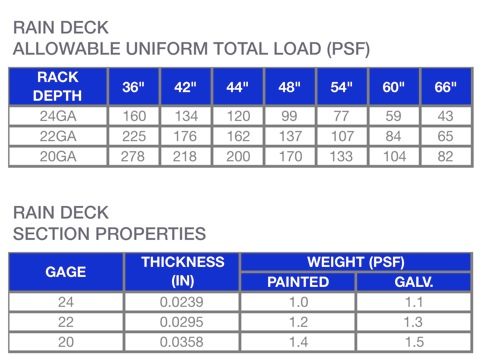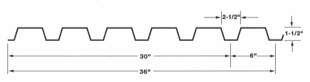Example Calculation:
A pallet rack level is 42” deep x 96” wide. What would be the lightest gage of RAIN DECK allowable to achieve a uniform storage level capacity of 4,000 pounds (LBS)?

Step 1 Determine the area (SF) of the storage level
42” x 96” = 4,032 SQ IN = 28 SF

Step 2 Divide the required capacity (4,000 LBS) by the area of the storage level (28 SF) to obtain the total load
4000 LBS/28 SF = 142.9 LBS/SF = 142.9 PSF

Step 3 Find the lowest number above 142.9 PSF in the 42” column and match the gage

Solution: 176 PSF = 22GA RAIN DECK